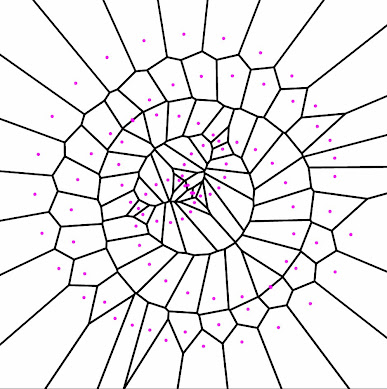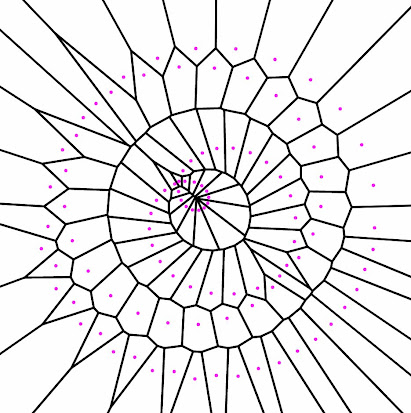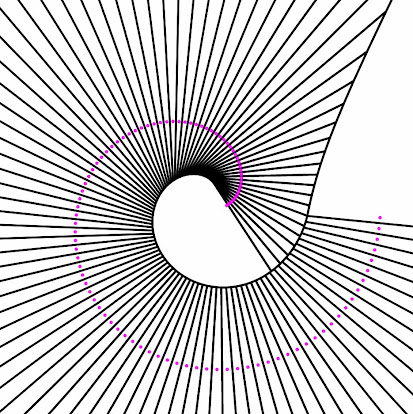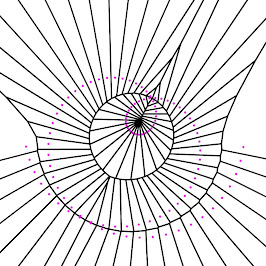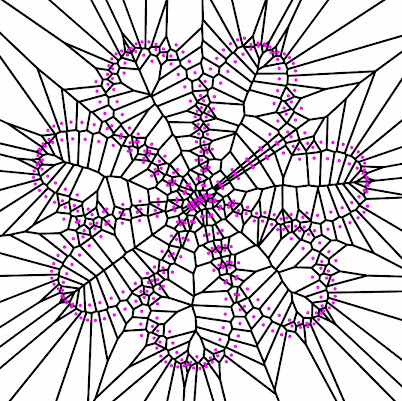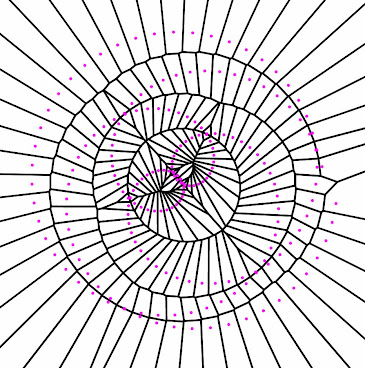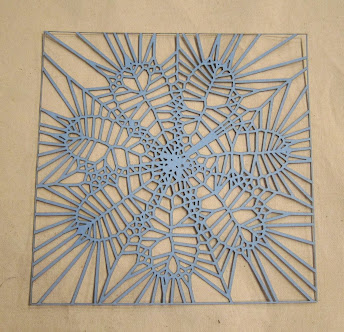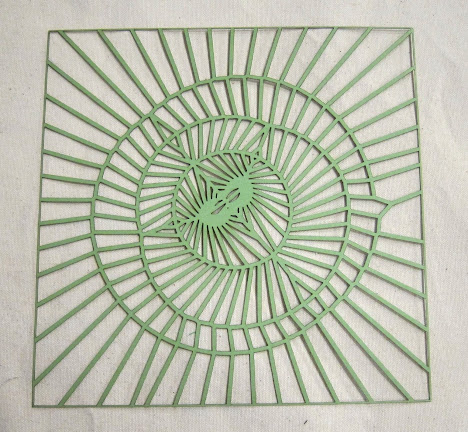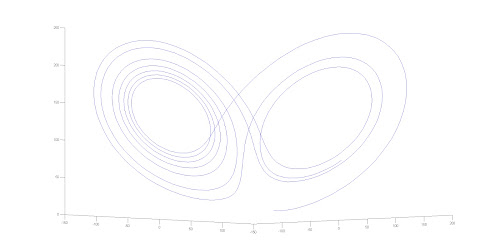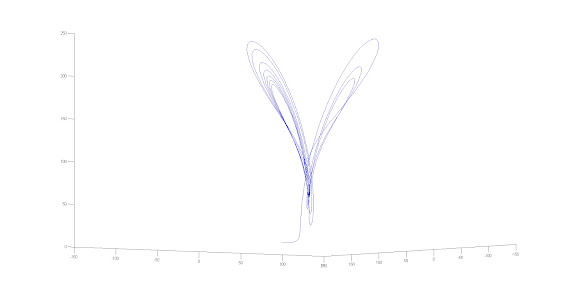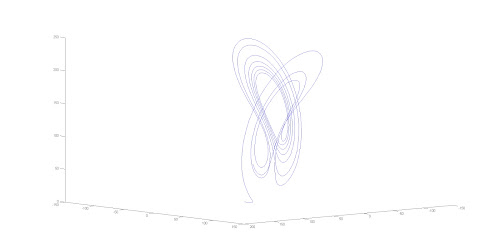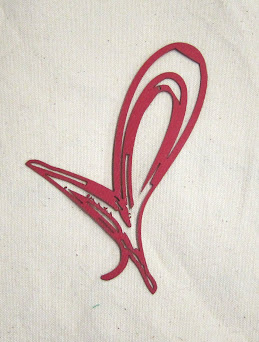### Rose Voronoi and Lorenz Attractor Designs

I made paper lace using a Voronoi diagram generated with a polar rose and a 2D graph of a Lorenz Attractor.

The polar rose is a the forms generated by the equation r= a*cos(theta*n/d) and results in the possible forms shown below (from Wikipedia). I chose this equation because it produces a large range of shapes from a single code.I inserted this equation in the the “Random” Voronoi generator by Jennifer. My code is below, but not properly commented.

void setup() {
size(600,600,P3D); //size of your intended pattern
noLoop(); // don’t need to use the draw loop
/*unique name for your file. if left unchanged,
will simply save file with current milisecond*/
String fileName= “voronoi”+millis()+”.pdf”;
beginRaw(PDF, fileName); //enables you to save your design to a pdf
setupVoronoi(); // create your voronoi generator
int petalLength = 250; // variable to control the maximum length of each petal
float n=.232; //this is n/d and determines the petal shape
int numPoints=100; //number of points plotted.
float theta = 0;
float r=0;
float x=0;
float y=0;
//this will draw one spiral
for (int k=1;k<numPoints;k++) {
theta = 2*3.14159/numPoints*k;//change first number to decide how many times around the circle are plotted.
r=petalLength*cos(n*theta);
x=r*cos(theta)+300;
y=r*sin(theta)+300;
}